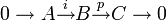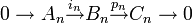# Short exact sequence of chain complexes gives long exact sequence of homology

## Statement

Suppose$A,B,C$ are chain complexes and we have a short exact sequence of chain complexes, i.e., a short exact sequence in the category of chain complexes with chain maps given by:$0 \to A \stackrel{i}{\to} B \stackrel{p}{\to} C \to 0$

Here, exactness means that for each integer$n$, the induced sequence of maps:$0 \to A_n \stackrel{i_n}{\to} B_n \stackrel{p_n}{\to} C_n \to 0$

is a short exact sequence of abelian groups (or modules, if this is a chain complex of modules).

Then, we can obtain (canonically) a long exact sequence of homology groups (respectively, modules):$\dots \stackrel{\operatorname{connecting}}{\to} H_n(A) \stackrel{H_n(i)}{\to} H_n(B) \stackrel{H_n(p)}{\to} H_n(C) \stackrel{\operatorname{connecting}}{\to} H_{n-1}(A) \stackrel{H_{n-1}(i)}{\to} H_{n-1}(B) \stackrel{H_{n-1}(p)}{\to} H_{n-1}(C) \stackrel{\operatorname{connecting}}{\to} \dots$

The maps from a given homology of$C$ to the lower homology of$A$ are connecting homomorphisms and arise via an application of the snake lemma.